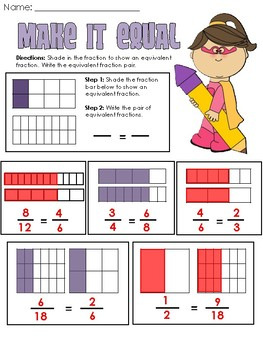DID YOU KNOW:
Seamlessly assign resources as digital activities

Learn how in 5 minutes with a tutorial resource. Try it Now

Learn More# Common Core: Equivalent Fractions PracticeGrade Levels
3rd - 5th
Subjects
Standards
Resource Type
Formats Included
• PDF
Pages
14 pages

### Description

The product includes six printable worksheets or activities for the students to complete as math center practice, homework, guided teacher center, exit cards, and more.

Each activity page allows the students more practice with identifying equivalent fractions and gaining a better understanding of equivalent fractions.

Students will have the opportunity to:
-Shade fraction bars to compare equivalent fractions
-Partition number lines to determine if fractions are equivalent
-Sort fraction circles that are equivalent
-Practice multiplying the numerator and denominator to create equivalent fractions

Please leave comments and feedback! I hope you enjoy using these activities in your classroom!
Total Pages
14 pages
Answer Key
Included
Teaching Duration
N/A
Report this Resource to TpT
Reported resources will be reviewed by our team. Report this resource to let us know if this resource violates TpT’s content guidelines.

### Standards

to see state-specific standards (only available in the US).
Recognize and generate simple equivalent fractions, (e.g., 1/2 = 2/4, 4/6 = 2/3). Explain why the fractions are equivalent, e.g., by using a visual fraction model.
Understand two fractions as equivalent (equal) if they are the same size, or the same point on a number line.
Explain equivalence of fractions in special cases, and compare fractions by reasoning about their size.

### Questions & Answers

Teachers Pay Teachers is an online marketplace where teachers buy and sell original educational materials.

More About Us